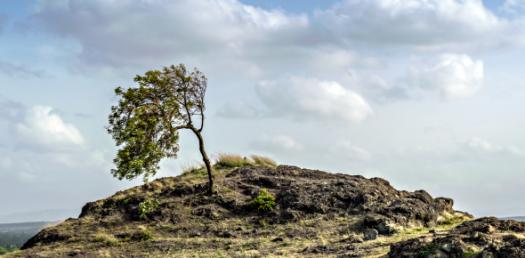# Velocity And Acceleration Quiz

5 Questions | Attempts: 5041
ShareSettingsIf you’re not studying physics, there’s a good chance that the differences between velocity and acceleration are lost on you – even if you drive a car or deal with them regularly. The term “velocity” refers to an object’s speed in a certain direction, whereas acceleration is the rate of change of that velocity with respect to time. See what else you can tell us about the two in this quiz!

• 1.
A group of bike riders took a 4.0-hour trip. During the first 3.0 hours, they traveled a total of 50. kilometers, but during the last hour they traveled only 10. kilometers. What was the group's average speed for the entire trip?
• A.

40. km/hr

• B.

30. km/hr

• C.

15 km/hr

• D.

60. km/hr

• 2.
One car travels 40. meters due east in 5.0 seconds, and a second car travels 64 meters due  east in 8.0 seconds. During their periods of travel, the cars definitely had the same
• A.

Total displacement

• B.

Average velocity

• C.

Average speed

• D.

Change in momentum

• 3.
The speed of a car is decreased uniformly from 30. meters per second to 10. meters per second in 4.0 seconds. The magnitude of the car's acceleration is
• A.

5.0 m/s2

• B.

10. m/s2

• C.

40. m/s2

• D.

20. m/s2

• 4.
A car having an initial velocity of 12 meters per second east slows uniformly to 2 meters per second east in 4.0 seconds. What is the acceleration of the car during this 4.0-second interval?
• A.

2.5 m/s2 west

• B.

2.5 m/s2 east

• C.

6.0 m/s2 east

• D.

6.0 m/s2 west

• 5.
Two cars, A and B, are 400. meters apart. Car A travels dueeast at 30. meters per second on a collision course withcar B, which travels due west at 20. meters per second. Howmuch time elapses before the two cars collide?1) 2) 8.0 s3) 20. s4) 40. s
• A.

13 s

• B.

8.0 s

• C.

20. s

• D.

40. s

## Related TopicsBack to top
×

Wait!
Here's an interesting quiz for you.